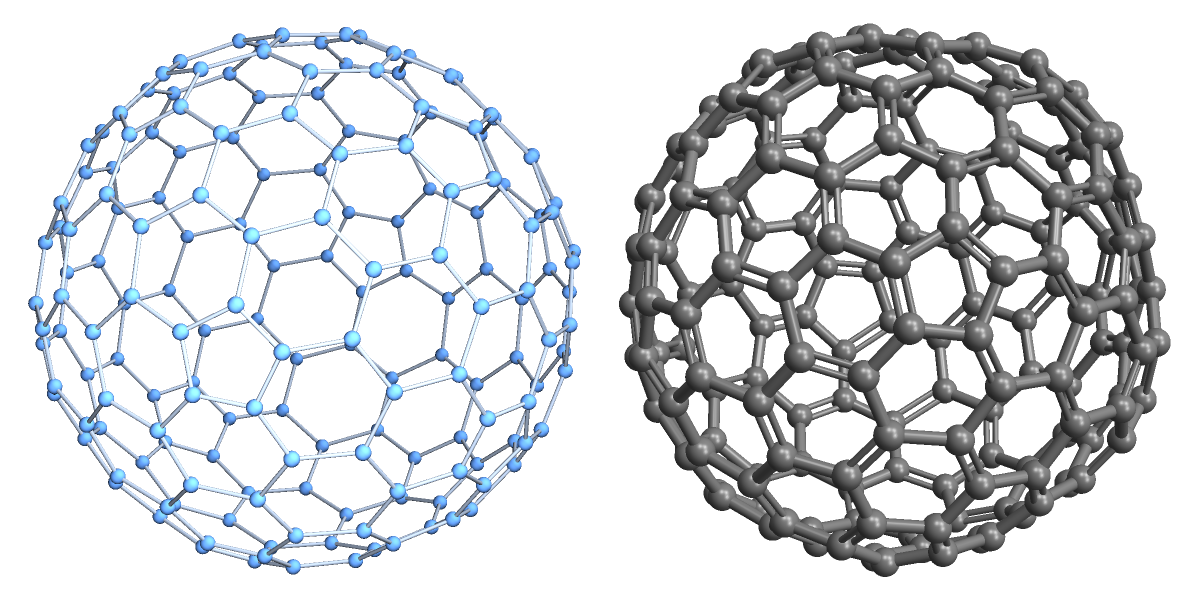#This resource function is obsolete. Use the function BuckyballGraph instead.

Function Repository Resource:

# BuckyballGraph

Create a graph of an order-n buckyball

Contributed by: Jan Mangaldan
 ResourceFunction["BuckyballGraph"][n] gives a graph corresponding to an order-n buckyball. ResourceFunction["BuckyballGraph"][class,n] gives a graph corresponding to an order-n buckyball of class class.

## Details and Options

Possible values of class include "I", "II", 1 and 2.
ResourceFunction["BuckyballGraph"][n] is equivalent to ResourceFunction["BuckyballGraph"][2, n].
ResourceFunction["BuckyballGraph"][1, n] or ResourceFunction["BuckyballGraph"]["I", n] generates a three-dimensional graph corresponding to the class I (n+1,0) Goldberg polyhedron, the dual polyhedron of a geodesic sphere.
ResourceFunction["BuckyballGraph"][2, n] or ResourceFunction["BuckyballGraph"]["II", n] generates a three-dimensional graph corresponding to the class II (n,n) Goldberg polyhedron.
ResourceFunction["BuckyballGraph"] takes the same options as Graph3D.
With the setting VertexCoordinates"Embedded", coordinates corresponding to the vertices of the buckyball are generated with a special method.

## Examples

### Basic Examples (3)

Generate an order-2 buckyball:

 In:=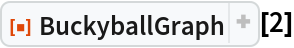Out=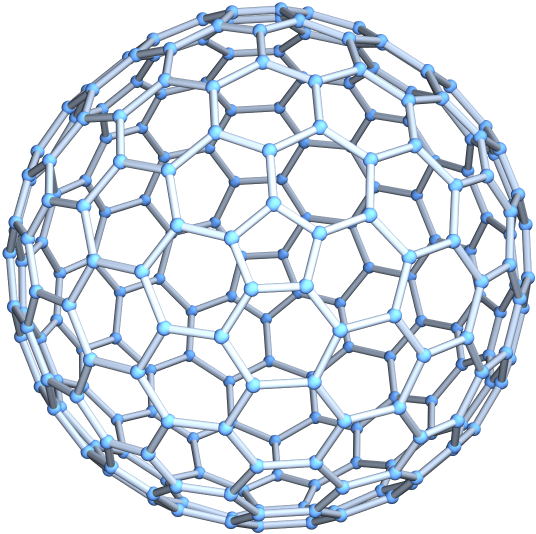The above buckyball is a class II, order-2 buckyball:

 In:=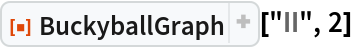Out=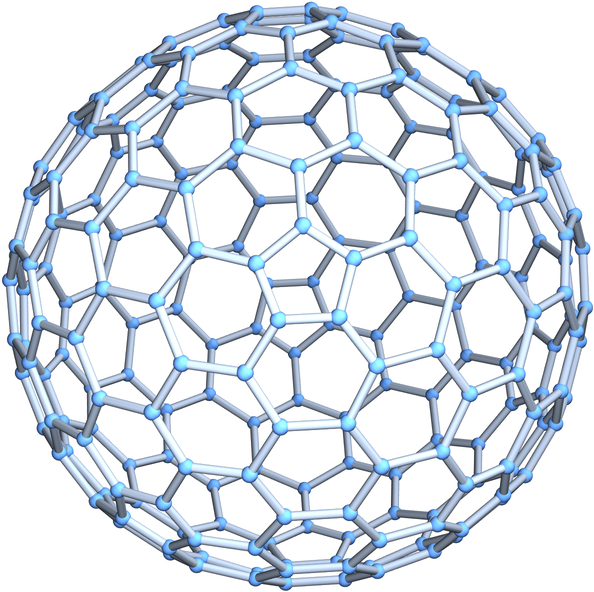Generate a class I, order-3 buckyball:

 In:=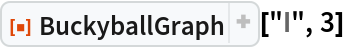Out=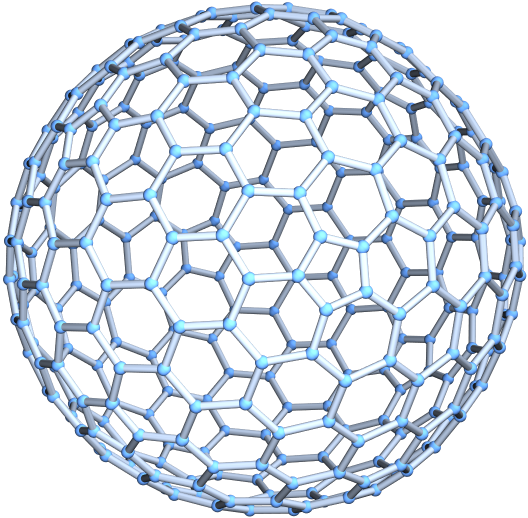Generate an order-2 buckyball with specially computed coordinates:

 In:=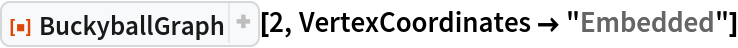Out=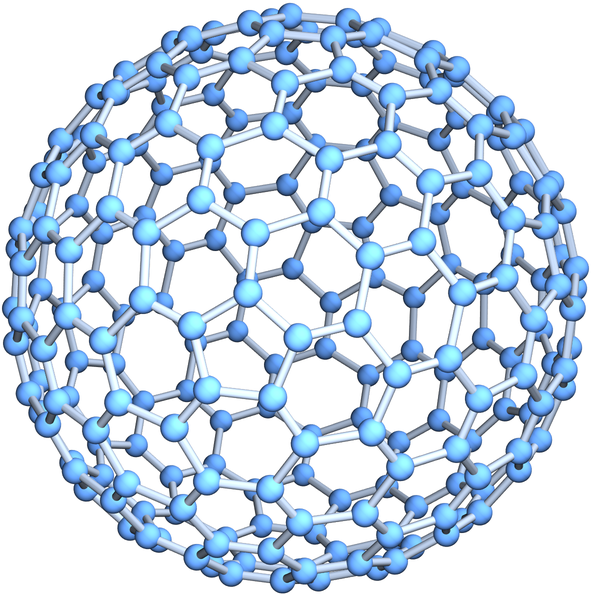### Scope (3)

Generate a dodecahedral graph:

 In:=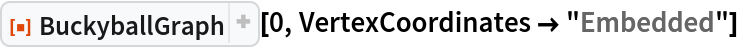Out=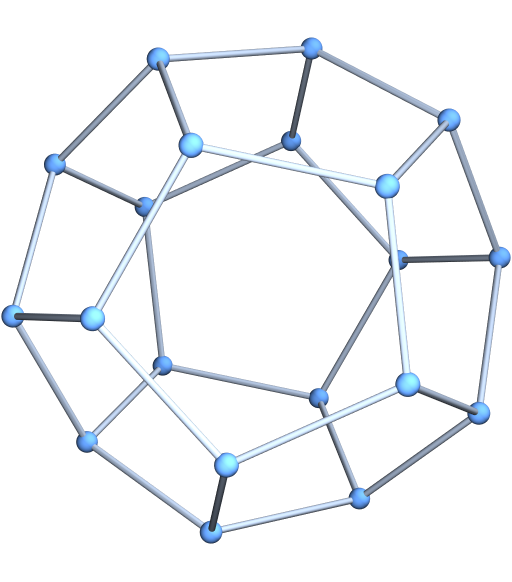Show an order-1 buckyball of different classes:

 In:=Out=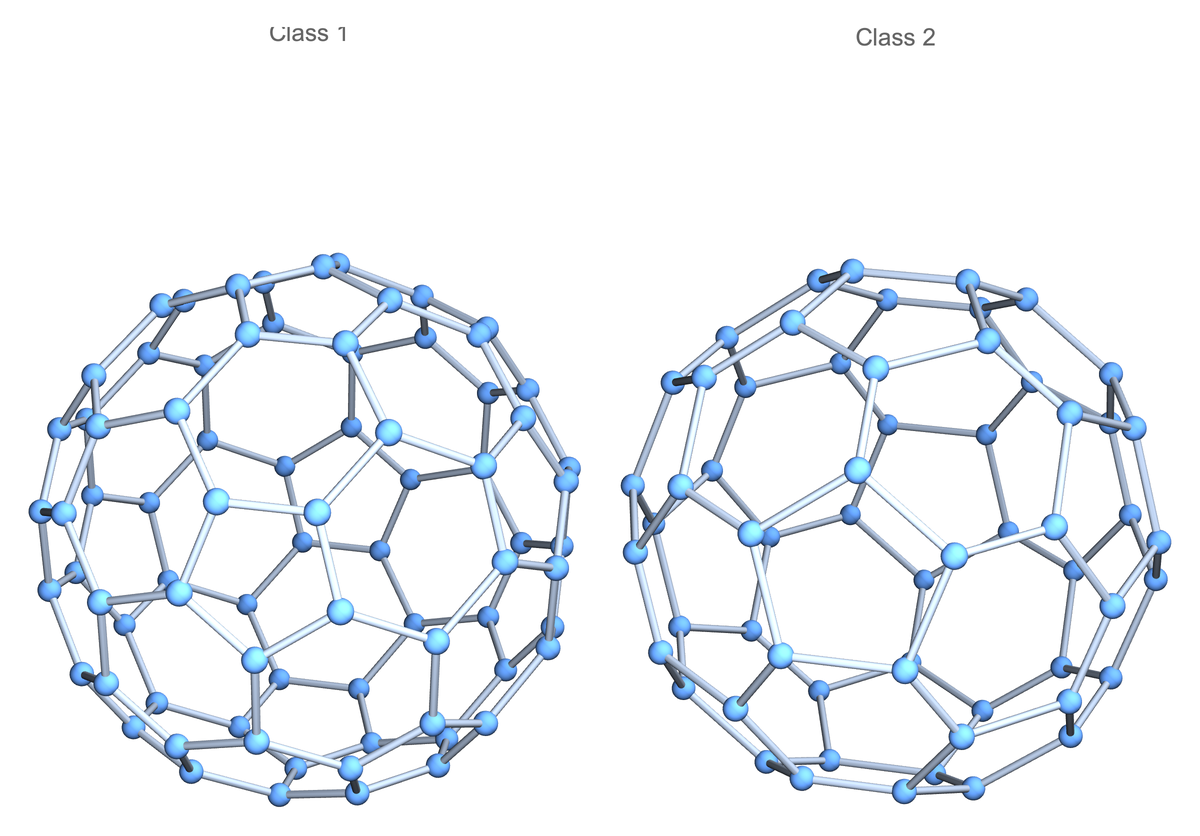Convert to a Graph object:

 In:=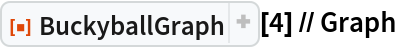Out=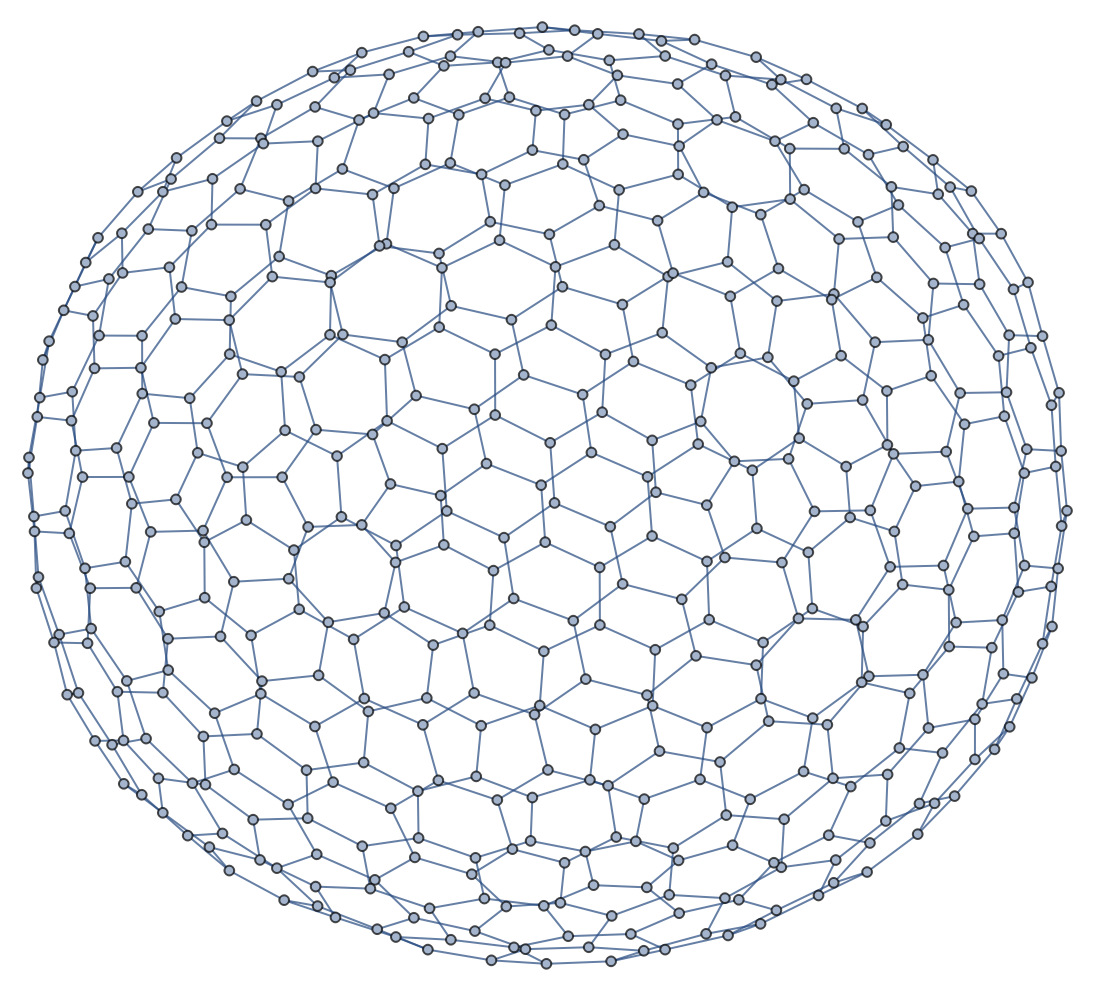### Options (4)

#### GraphLayout (1)

Specify various layouts for the buckyball graph:

 In:=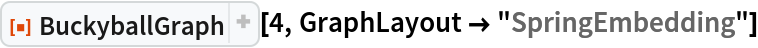Out=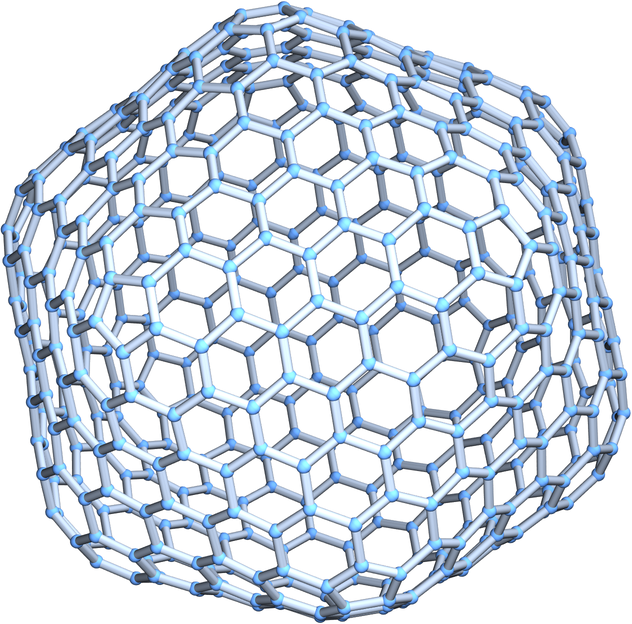In:=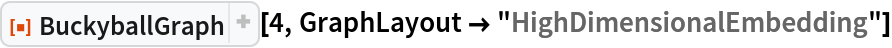Out=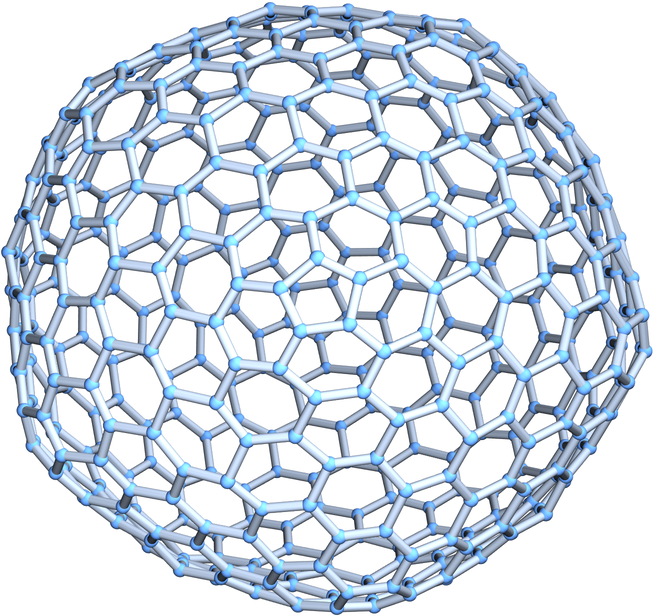#### PlotTheme (1)

Use a large graph theme:

 In:=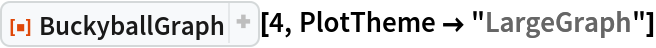Out=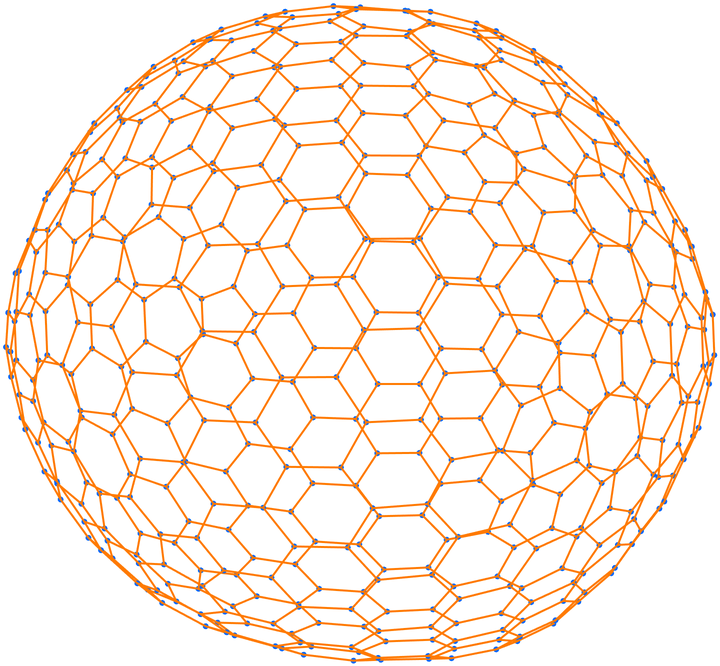#### VertexCoordinates (2)

By default, vertex coordinates are computed automatically, depending on the setting for GraphLayout:

 In:=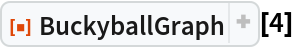Out=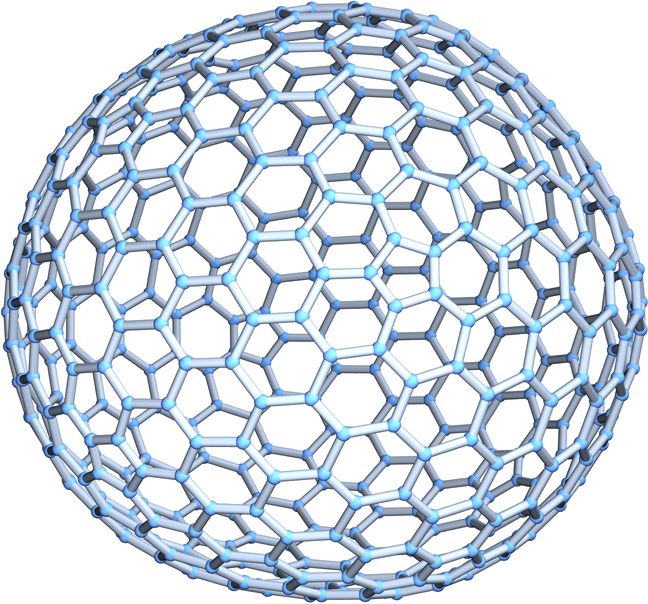Use specially computed coordinates for the vertices:

 In:=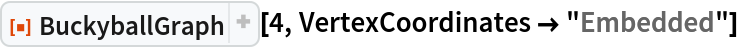Out=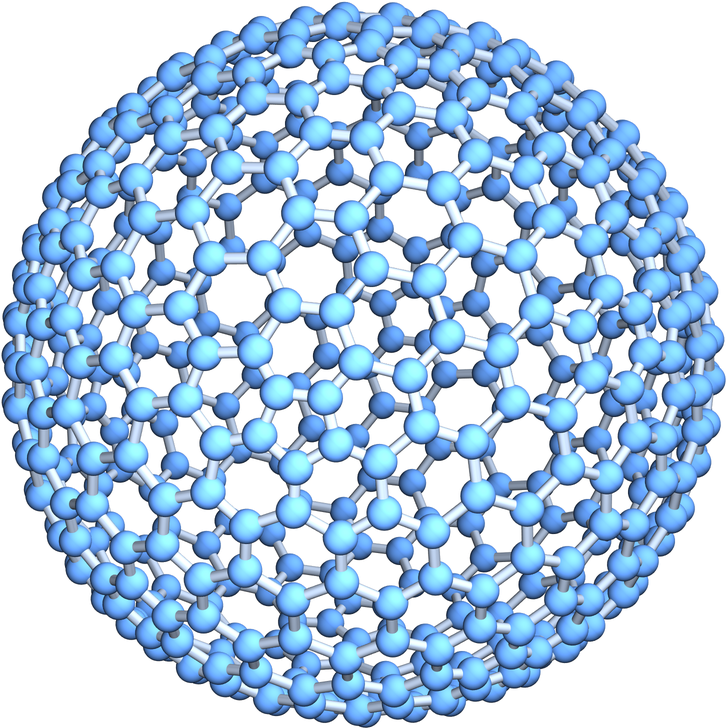### Properties and Relations (1)

BuckyballGraph (the class II, order-1 buckyball) is the graph corresponding to the truncated icosahedron:

 In:=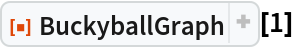Out=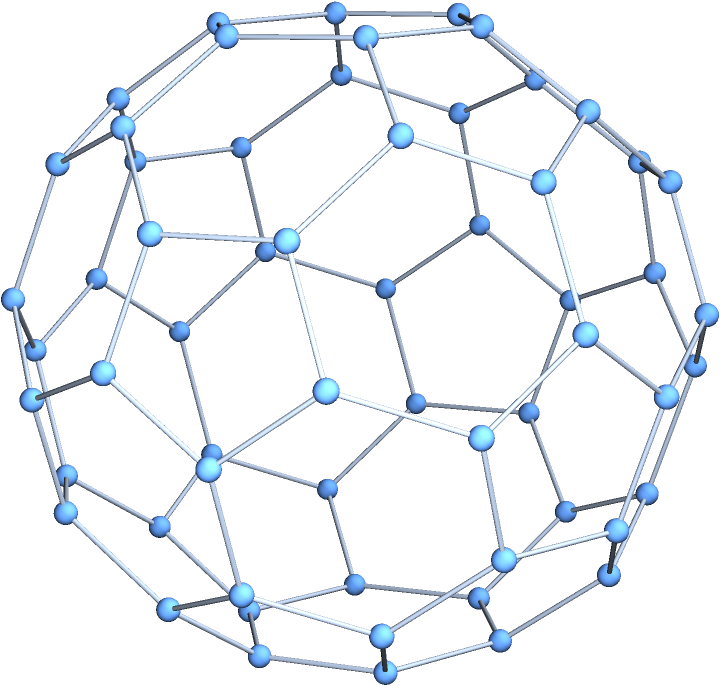In:=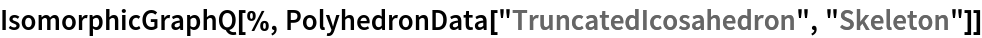Out=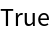### Neat Examples (2)

Generate a fullerene molecule from its corresponding skeletal graph:

 In:=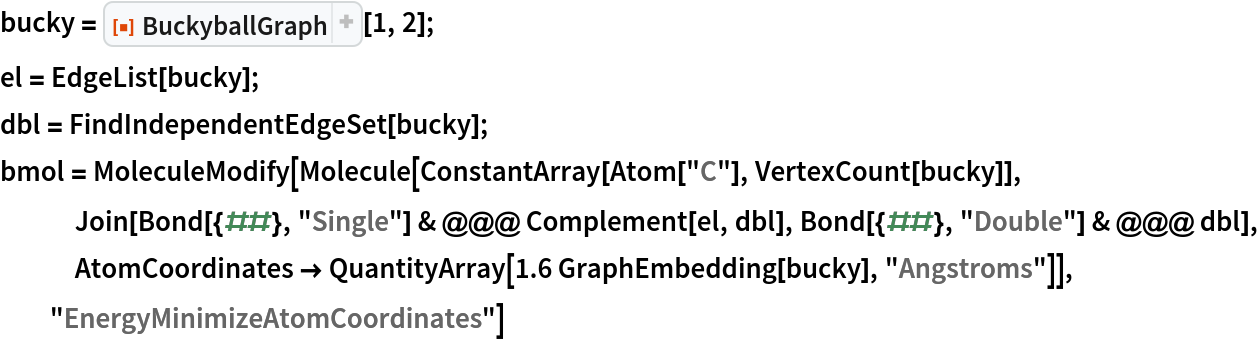Out=Visualize the graph and the corresponding fullerene:

 In:=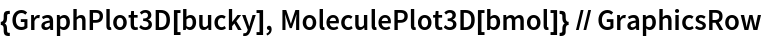Out=